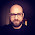## Friday, August 22, 2014

### Javascript and Fuzuli Integration

JFuzuli, the Java implementation of Fuzuli Programming Language now supports limited Javascript integration.

JFuzuli currently supports passing Fuzuli variables to Javascript environment, passing Javascript variables to Fuzuli environment, embedding Javascript code in any part of a Fuzuli source code.

The full support is planned to have ability of calling Fuzuli functions directly from within Javascript.

Here is the examples. This is the simplest one to demonstrate the basic usage of Javascript support:

In the example above, the variable a is set to 10 in Fuzuli part, is incremented by 1 in Javascript part and is printed in the Fuzuli part again. After all, value of a is 11.

In the example above, the variable message is first defined in Javascript section and was null in Fuzuli section at the top. And also, it is clear that the variable message is defined using the var keyword in Javascript section. After all, at the Fuzuli section, message is printed with its value which was set in Javascript section.

The example above is more interesting as it has a function which is written in Fuzuli language, but the function has its body written in Javascript! In this example, square function has a single parameter x. x is then passed to Javascript body and the result is calculated. Value of result is then returned in Fuzuli. At the end, the Fuzuli function call  (square 5) simply returns 25 which is calculated by Javascript.

Passing Arrays

Because the list object in Fuzuli is simply a java.util.ArrayList, all public fields and methods of ArrayList are directly accessable in Javascript section. Look at the example below. In this example a list object is created with values 1,2 and 3, respectively. In Javascript section, the values of this object is cleaned first and then 10 and 20 are added to the list. Finally, in the Fuzuli section, object is printed only with values 10 and 20.

List objects can be created directly in Javascript section. Look at the example below. Since JFuzuli interpreter uses the javax.scripting framework, a Java object can be created with new keyword. The variable a is a list object in Fuzuli section again and the printed output includes two values of 10 and 20.

You can try similar examples using our online interpreter in url

http://fuzuliproject.org/index.php?node=tryonline

Hope you get fun with Fuzuli...

1.2.